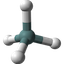What Is Molecular Formula

Q

What Is Molecular Formula?

✍: FYIcenter.com

AA Molecule Formula is an expression of element symbols and numbers of atoms of same element types that bonded together to form the molecule. If the number of atoms of an element type is 1, it is omitted from the formula. If the number of atoms of an element type is greater than 1, it is expressed as a subscript following the element symbol.

Examples of molecule formulas:

• Molecule formula for Dioxygen is O2.
• Molecule formula for Water is H2O.
• Molecule formula for Carbon Dioxide is CO2.
• Molecule formula for Salt is NaCl.
• Molecule formula for Sugar is C6H12O6.

Â

â‡‘â‡‘ Molecule FAQ

2020-04-16, 667👍, 0💬